### Home > PC > Chapter 6 > Lesson 6.3.1 > Problem6-113

6-113.
1. Given ΔHAT where ∠H = 25° and HA = 10. 6-113 eTool (Desmos) Homework Help ✎

1. What is a possible length of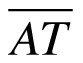that would make two triangles? Sketch a diagram of the triangle to demonstrate.

2. What are the possible lengths forthat will make exactly one triangle?

3. For what values ofwill not form a triangle?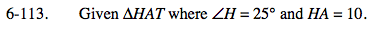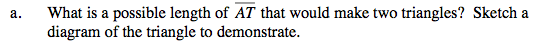Use the eTool below to visualize this.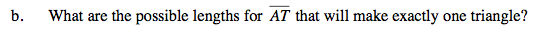The ⊥ distance to HT . Calculate it.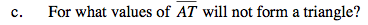Shorter than the ⊥ distance to HT .